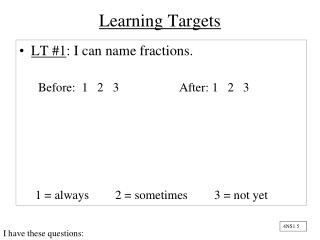DownloadDownload PresentationLearning Targets

# Learning Targets

Download Presentation## Learning Targets

- - - - - - - - - - - - - - - - - - - - - - - - - - - E N D - - - - - - - - - - - - - - - - - - - - - - - - - - -
##### Presentation Transcript

1. Learning Targets • LT #1: I can name fractions. Before: 1 2 3 After: 1 2 3 1 = always 2 = sometimes 3 = not yet 4NS1.5 I have these questions:

2. Learning Targets • LT #2: I can find the least common denominator (also knows as the least common multiple). Before: 1 2 3 After: 1 2 3 1 = always 2 = sometimes 3 = not yet I have these questions: 6NS2.4

3. LT #1 Naming Fractions A Fraction Shows -> Numerator Denominator Part Whole Name the Fraction shown by the shaded part: Name the fraction shown by the un-shaded part 2 3 5 5

4. Multiple The product of a whole number multiplied times any other whole number. Name the first six multiples of 6 6, 12, 18, 24, 30, 36

5. Name the first six multiples of 5 5, 10, 15, 20, 25, 30

6. Common Multiple • A Common Multiple is a number that is a multiple of two numbers. • Here are common multiples for the numbers 4 and 10 4 = 4, 8, 12, 16, 20, 24 10 = 10, 20, 30, 40, 50,

7. Least Common Multiple Smallest number that a set of given numbers divides evenly into. LCM - Least Common Multiple - LCM is also known as finding the Least Common Denominator It is useful to know how to do this so you can find a common denominator when comparing, adding or subtracting fractions.

8. Least Common Multiple TO FIND THE LCM OF TWO NUMBERS • List the multiples of both numbers • Find the least multiple that • both numbers have in common.

9. What is the Least Common Multiple of 6 & 8 6 = 6, 12, 18, 24, 30, 36 8 = 8, 16, 24, 32, 40, 48

10. Find the least common multiple for: 4 and 22

11. Find the least common multiple for 8 and 12.

12. THE END! Take out your Learning Targets.

13. LT #1 Naming Fractions A Fraction Shows -> Numerator Denominator Part Whole Name the Fraction shown by the shaded part: Name the fraction shown by the un-shaded part 2 3 5 5

14. LT #2 Least Common Denominator • 1) Findthemultiples of both numbers TO FIND THE LCM OF 4 and 12: 4 = 4, 8, 12, 16, 20… 12 = 12, 24, 36… 2)Find the least (smallest) multiple that the numbers have in common. LCM is 12 Least Common Multiple is also known as LCD…the “D” standing fordenominator

15. Name___________October 15, 2012 Period___ Statues and Snakes Pizzazzies